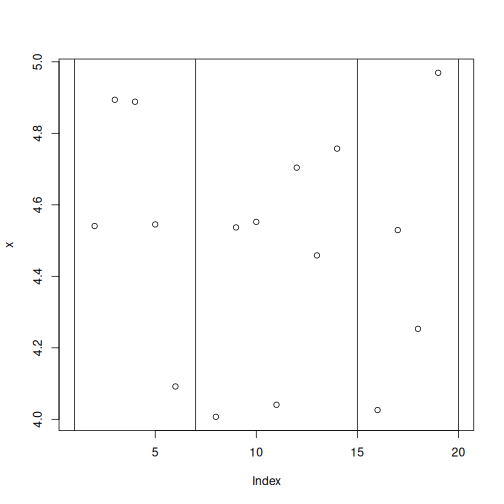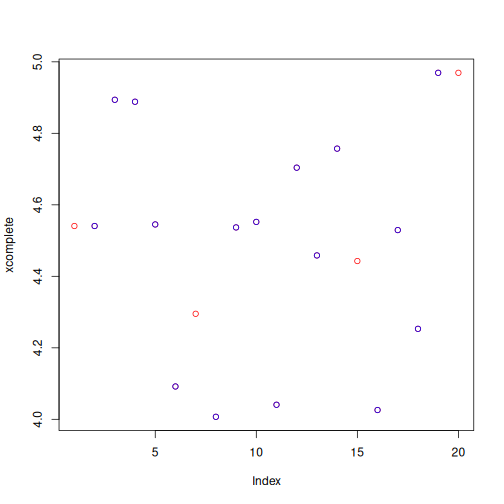# Example

1. Data completion protocol :

``````repDonnees="~/Protocole_uHMM/Protocole_NA"
``````
An example of this protocol with an artificial dataset is realized. (see Completion tab). The data are created randomly with the only constraint the dispersion of points between 4 and 5. The addition of missing values (NA= Not Available) is voluntary.

The data series is named "x" and is plotted. The vertical lines represent the locations of the NA.

``````x=rep(4,16)+runif(16,0,1)
x=c(NA,x[1:5],NA,x[6:12],NA,x[13:16],NA)
indNA=which(is.na(x))
``````The NA indexes (Not Available=missing value) of the x dataset are located, and put in the vector "indNA".

``````indNA=which(is.na(x)); indNA
``````
``````##   1  7 15 20
``````

The total number of NA value is calculated, this corresponds to the length of the vector "indNA".

``````nb.indNA=length(indNA); nb.indNA
``````
``````##  4
``````

The total number of value is calculated, this corresponds to the total length of "x".

``````nb.x=length(x); nb.x
``````
``````##  20
``````

Duplication of "x" dataset to "xcomplete" is performed to avoid overwriting the raw data.

``````xcomplete=x
``````

A "for" loop is performed to execute an average. This average is calculated from the 2 values before and the 2 values after the missing value.

2. remarks :

• If the missing value is the 1st value in the series, then it takes the value of the next data, if it is not also a missing value.

• If the missing value is the last in the series, then it takes the value of the data that precede, if it is not also a missing value.

``````delai=2
for(i in 1:nb.indNA){
val=indNA[i];
if(val<=delai){xcomplete[val]=x[val+1];#copie du suivant
}else{
if(val>=(nb.x-delai)){xcomplete[val]=x[nb.x-1]; #copie du precedent
}else{
borneMin=max(1,val-delai); #les 2 valeurs avant le NA, avec comme minimum le début de la fenêtre à 1
borneMax=min(val+delai,nb.x); #les 2 valeurs après le NA, avec comme maximum la fi de la fenêtre égale à la longueur de la série.
if (sum(is.na(x[borneMin:borneMax]))<(borneMax-borneMin+1)){
xcomplete[val]=mean(x[borneMin:borneMax],na.rm=T);
}
}
}
}
``````